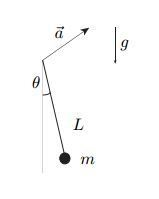# Accelerated mathemathical pendulum

## Homework Statement

Mathemathical pendulum with mass ##m## on a line ##L## is moving with constant acceleration in xz plane, ##\vec{a}=(a_x,a_z)##. Let's consider only the oscillations in xz plane. Find stationary value of ##\theta ## and find the frequency of small movements around the stationary angle if ##a_x=0##.## The Attempt at a Solution

Ok, let's put one coordinate system somewhere :D and another one on the top of line ##L##. Let's say that these two coordinate systems are connected with vector R.

Let's express everything in accelerated system:

##\vec{r}=(Lsin\theta , Lcos\theta )## and ##\vec{R}=(-\frac{1}{2}a_xt^2,-\frac{1}{2}a_yt^2)##

Both together give me ##v=(\dot{\theta }Lcos\theta -a_xt,-\dot{\theta }Lsin\theta -a_yt)## and ##v^2=\dot{\theta }^2L^2+t^2(a_x^2+a_y^2)+2\dot{\theta }Lt(sin\theta a_y-cos\theta a_x##

So ##L=T-V=\frac{1}{2}m(\dot{\theta }^2L^2+t^2(a_x^2+a_y^2)+2\dot{\theta }Lt(sin\theta a_y-cos\theta a_x)+mgLcos\theta ##.

I won't even write the equations from here one because this brings me to wrong simple solution:

##\ddot{\theta }+\frac{g}{l}\theta =0## which can not be ok. It looks like I am missing something when calculating the velocity? But what?

## Answers and Replies

Simon Bridge
Science Advisor
Homework Helper
I'd have tried:
In the accelerated frame, rotate the axis to reflect the apparent direction of gravity - you should end up with the same relations as you are used to right?

To recover the problem-statement x and y directions, transform back.

Note: I originally interpreted the diagram as indicating that the pivot was subject to an applied force.

So ##L=T-V=\frac{1}{2}m(\dot{\theta }^2L^2+t^2(a_x^2+a_y^2)+2\dot{\theta }Lt(sin\theta a_y-cos\theta a_x)+mgLcos\theta ##.

I won't even write the equations from here one because this brings me to wrong simple solution

That's the problem. If I would write everything one of you would for sure notice that I was not careful.

Anyway, calculating everything ok brings me to

##\ddot{\theta }+\frac{1}{L}(a_xcos\theta +a_ysin\theta +g sin\theta )=0##

And therefore stationary angle ##\theta _0=arctg(\frac{a_x}{a_y+g})##

And for ##a_x=0## also

##\ddot{\theta }+\frac{1}{L}(a_y+g)\theta =0##